# Rectangle vs square

The rectangle has dimensions of 13 × 10, square 8 × 8. Which shape has more area and how much above?

Result

Δ S =  66

#### Solution:Leave us a comment of example and its solution (i.e. if it is still somewhat unclear...):Be the first to comment!## Next similar examples:

1. Square plate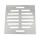From a square metal plate with a side long 6dm were cut two identical rectangular openings with dimensions of 2.5 dm and 2 dm. Calculate the remainder of the square plate.
2. Square to rectangle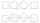What is the ratio of the area of a square of side x to the area of a rectangle of a rectangle of width 2 x and length 3
3. Photo frameA square shape photo with a side length of 20 cm is framed by a 4 cm wide bar. Find the external frame size of this photo (the frame is from all sides)
4. Rectangular fieldOne dimension of the rectangular field is 56 m greater than second dimension. If each side of the rectangle increases by 10 m, increases the surface field is 1480 m2 . Determine dimencions of the field.
5. A rectangleA rectangle has an area of 36 cm2. What could the length and width of rectangle be?
6. RectangleDraw a rectangle with the sides a = 4 cm, b = 5 cm. Mark the center of symmetry S and all axes of symmetry. How many axes of symmetry has? Write down.
7. Area of squareCalculate the content area of the square whose perimeter is 24 dm.
8. SquaresCalculate the area of square and result round to square decimeters. a) a = 1,52 dm b) a = 13 268mm c) a = 562 cm d) a = 1,52 m
9. Garden plotCalculate how many meters of fence need to fence the square garden with length and width of 22 meters.
10. Strip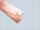From 5.9 cm wide strip should be cut rhombus with area 28 cm2. How long will be its side?
11. DoctorsIn the city operates 171 doctors. The city has 128934 citizens. How many citizens are per one doctor?
12. Roman numerals 2+Add up the number writtens in Roman numerals. Write the results as a roman numbers.
13. Compare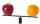Compare with characters >, <, =: 85.57 ? 80.83
14. To thousands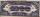Round to thousands following numbers:
15. ProductResult of the product of the numbers 1, 2, 3, 1, 2, 0 is:
16. Roman numeralsWrite numbers written in Roman numerals as decimal.Added together and write as decimal number: LXVII + MLXIV